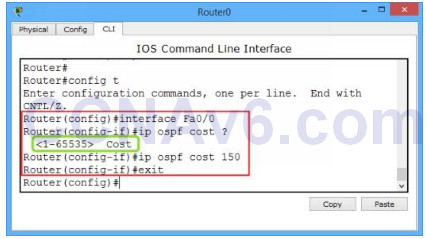120 Labs for Cisco CCNA 200-125 and CCENT Exams

# Lab 110: Configuring OSPF Cost Metric

In the previous exercise, we have discussed basics of the Open Shortest Path First (OSPF) protocol and how to configure OSPF routing. In this lab exercise, we will explain how the OSPF protocol decides the best (or shortest) path to a destination network and how to configure OSPF cost metric value.

The OSPF routing protocol uses the cost metric to determine the best path to a destination network. The cost metric is based on the bandwidth of interfaces. The total cost of a route is equal to the sum of all outgoing interface costs. The lowest cost is preferred to route the network traffic.

The following table lists the default OSPF cost values used by Cisco routers, which depends on the types of interface being used for a specific route.

 Sr. No. Interface Type Bandwidth Cost Value 1 Serial 56 Kbps 1562 2 T1 1.544 Mbps 64 3 Token Ring 4 Mbps 25 16 Mbps 6 4 Ethernet 10 Mbps 10 5 Fast Ethernet 100 Mbps 1

#### How to calculate OSPF cost?

Now, let’s have a look how the OSPF cost metric is calculated. To calculate the OSPF cost metric of an interface, the following formula is used.

• Cost = 100/ the bandwidth of the interface in Mbps

1.  For example, let’s calculate the OSPF cost metric of an Ethernet interface. We know that the bandwidth of an Ethernet interface is 10.

• Cost = 100/ 10 = 10

2.  Let’s have a look at another example: how to calculate the cost of a T1 interface?

• Cost = 100/ 1.544 = 64 (approximately)

Note: For the Serial interfaces, OSPF will use the configured bandwidth, measured in Kbps, to determine the cost.

#### How to change the default OSPF cost metric?

You can change the default cost metric value for an interface. However, you should be careful otherwise your network may misbehave if you have configured the cost metric value inappropriately. In order to change the default OSPF cost metric value, execute the following command.

```Router( config)# interface < name/ number >
Router( config-if)# ip ospf cost < cost value 1-65535 >```

For example, let’s set the OSPF cost metric value for the interface Fa0/ 0 as 150. To do so, execute the following command.

```Router( config)# interface Fa0/ 0
Router( config-if)# ip ospf cost 150```

The following figure shows how to change the default OSPF cost metric.### Related Articles

Subscribe
Notify ofThis site uses Akismet to reduce spam. Learn how your comment data is processed.